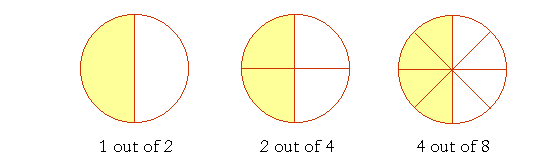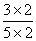Definition Of Equivalent Fractions

Equivalent Fractions of a fraction are those fractions whose numerator and denominator are in the same ratio as that of the original fraction.

Equivalent fraction can be generated by multiplying or dividing the numerator and denominator by the same number.Example of Equivalent Fractions

In the given figure, shaded parts of the figures represent the equivalent fractions as 1/2 = 2/4 = 4/8.

Solved Example on Equivalent Fractions

Ques: Victor had 5 chocolates. He gave 2 chocolates to his friends. Which of the following are the two equivalent fractions for the fraction of the chocolates left with Victor?

Choices:

A. 6/10and 9/15
B. 3/15 and 4/20
C. 2/10 and 3/15
D. none of the above
Step 2: The fraction of the chocolates left with Victor =Step 4:= 6/10 [Multiply both numerator and denominator by the same non-zero number, 2.]
Step 5:[Multiply both numerator and denominator by the same non-zero number, 3.]